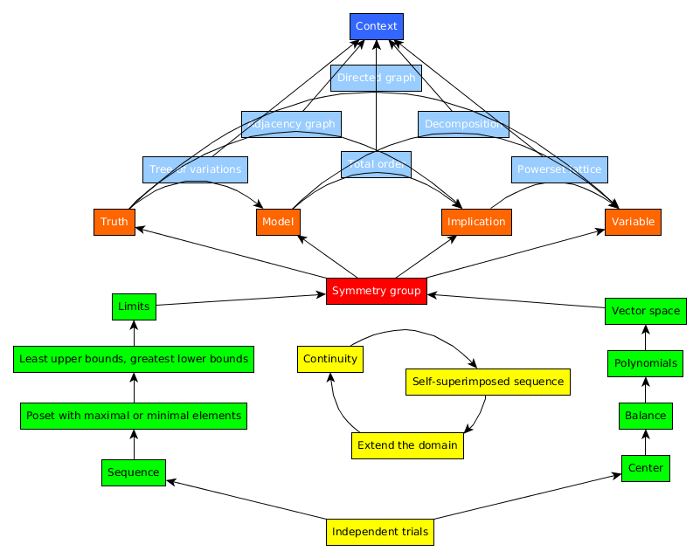• ms@ms.lt
• +370 607 27 665
• My work is in the Public Domain for all to share freely.

Introduction E9F5FC

Understandable FFFFFF

Questions FFFFC0

Notes EEEEEE

SoftwareHow does the three-cycle extend our existing mathematical language?

Zermelo-Fraenkel axioms of set theory

• Axiom of Extensionality. Two sets are the same set if they have the same elements.
• Axiom of Regularity. Every non-empty set x contains a member y such that x and y are disjoint sets. This implies, for example, that no set is an element of itself and that every set has an ordinal rank.
• Axiom Schema of Specification. The subset of a set z obeying a formula ϕ(x) with one free variable x always exists.
• Axiom of Pairing. If x and y are sets, then there exists a set which contains x and y as elements.
• Axiom of Union. The union over the elements of a set exists.
• Axiom Schema of Replacement. The image of a set under any definable function will also fall inside a set.
• Axiom of Power Set. For any set x, there is a set y that contains every subset of x.
• Well-Ordering Theorem. For any set X, there is a binary relation R which well-orders X.

Also:

• Axiom of infinity. Let S(w) abbreviate w ∪ {w}, where w is some set. Then there exists a set X such that the empty set ∅ is a member of X and, whenever a set y is a member of X, then S(y) is also a member of X.

Implicit math: Sets are simplexes. Are simplexes defined by their subsimplexes as well?

• Well-ordering theorem. Each vertex is related by edges to the other vertices. Established by the q-weight.
• Axiom of power set. The power sets are the lattice paths in Pascal's triangle.
• Axiom of union. Simplexes combine to form larger simplexes.
• Axiom of pairing. Simplexes can be "collapsed" or "represented" by a vertex, the highest vertex. Two vertices are linked by an edge.
• Axiom of regularity.
• Axiom of extensionality. Simplexes are defined by their vertices. And the edges?

Eightfold way

• Axiom of Pairing. If x and y are sets, then there exists a set which contains x and y as elements.
• Axiom of Extensionality. Two sets are the same set if they have the same elements.
• Axiom of Union. The union over the elements of a set exists.
• Axiom of Power set. For any set x, there is a set y that contains every subset of x.
• Axiom of Regularity. Every non-empty set x contains a member y such that x and y are disjoint sets.
• Well-ordering theorem. For any set X, there is a binary relation R which well-orders X.
• Axiom Schema of Specification. The subset of a set z obeying a formula ϕ(x) with one free variable x always exists.
• Axiom Schema of Replacement. The image of a set under any definable function will also fall inside a set.

Reorganizings

• Evolution. Tree of variations. Axiom of Pairing (subbranches are part of a branch).
• Atlas. Adjacency graph. Axiom of Extensionality. Two levels of equality.
• Handbook. Total order. Well-Ordering Theorem.
• Chronicle. Powerset lattice. Axiom of Power Set.
• Catalog. Decomposition. Axiom of Union.
• Tour. Directed graph. Axiom of Regularity.

Relate to multiplicationNotes

Total order is the same as a labeled simplex.

Extension: 3! + (4 + 4 + 4 + 6) = 4!

3! = 2! + 4 (representations: 2 for edge and 4 for vertex)

We may assign the weight q^(k-1) to the kth vertex and the weights 1/q to each new edge. These weights give each vertex a unique label. The weight of each k-simplex is then the products of the weights of their vertices and edges. The Gaussian binomial coefficients count these weighted k-simplexes. Without the weights the vertices are distinct but there is no way to distinguish them. The symmetry group is the Symmetric group which relabels the vertices.

Matematikos išsiaiškinimo būdai

#### MathDiscovery

Naujausi pakeitimai

 Puslapis paskutinį kartą pakeistas 2019 liepos 17 d., 17:49